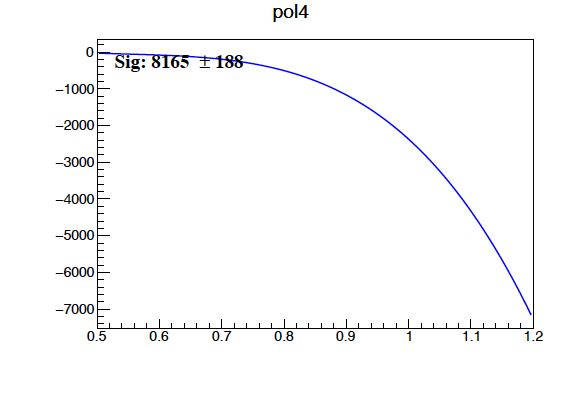# Issue to draw a result from fitting using TF1NormSum

Hi all,
I am using TF1NormSum to fit my data. The result of the fitting looks fine as you see below. I am trying to draw the 2 functions that I used but they show as a line as you see in the attached picture. I have copied the part that I did to draw the functions. Is there something wrong that I did?

``````****************************************
Chi2                      =      161.695
NDf                       =           46
Edm                       =  1.94849e-06
NCalls                    =         2152
NSig                      =      39031.7   +/-   390.32
Nbkgd                     =      47021.2   +/-   408.417
Mean                      =        0.939                      	 (fixed)
Sigma                     =     0.035457   +/-   0.00030317   	 (limited)
Alpha                     =    -0.523113   +/-   0.0103072    	 (limited)
N                         =      16304.6   +/-   1842.89      	 (limited)
p0                        = -1.67539e+06   +/-   73357.9
p1                        =  5.24474e+06   +/-   156862
p2                        =  3.17266e+06   +/-   177887
p3                        = -2.32717e+07   +/-   533497
p4                        =  1.87577e+07   +/-   348942
``````

``````# ++++ Plot Signal curve +++++++
f_crys.SetLineColor(kMagenta)
for i in range(0,f_crys.GetNpar()):
f_crys.SetParameter(i,f_sum.GetParameter(i+2))
integral = f_crys.Integral( 0.5, 1.2)
f_crys.SetParameter(0, f_sum.GetParameter(0) / integral)
f_crys.Draw("same")
print(f_sum.GetParameter(0) / integral)

# ++++ Plot bkgd curve +++++++
f_bc.SetLineColor(kBlue)
for i in range(0,f_bc.GetNpar()):
f_bc.SetParameter(i,f_sum.GetParameter(i+5))
f_bc.Draw("same")
``````

Maybe @couet can take a look

Can you provide a script plotting the magenta curve ?

here is the code I used. The background function looks fine and I can draw it. But I still have issue with the amplitude of Crystal ball function.

``````nsig = 50000
nbkg = 1000000
nEvents = nsig + nbkg
nBins = 1000

signal_mean = 3.0

f_crys = ROOT.TF1("f_crys", "crystalball", 0.5, 1.2)
f_bc = ROOT.TF1("f_bc", "pol4", 0.5, 1.2)

for i in range(1, 2):
can.cd(1)

h_sum = hhs.get('detMM_passdcxdcyRmincut_Pmmbin'+str(i))
f_crys.SetParameters(1000, 0.939, 0.03541, -0.5252, 2000)
f_bc.SetParameters(1, 1, 1, 1, 1)
# need to scale histogram with width since we are fitting a density
h_sum.Draw('')
print(i,' ', Pmm_bins[i], ' ', Pmm_bins[i+1])
h_sum.SetTitle('#scale{#bf{#font{P_{mm} = [}}} '+str(Pmm_bins[i])+' - '+str(Pmm_bins[i+1])+'#scale{#bf{#font{ ]}}}')

h_sum.SetXTitle('#scale{#bf{#font{ MM [GeV]}}} ')
h_sum.SetLineWidth(2)
h_sum.GetXaxis().SetTitleOffset(1.3);
h_sum.GetXaxis().SetTitleSize(0.05);
h_sum.GetXaxis().SetLabelSize(0.05);
h_sum.GetXaxis().SetLabelFont(22);

h_sum.GetYaxis().SetTitleOffset(1.2);
h_sum.GetYaxis().SetTitleSize(0.05);
h_sum.GetYaxis().SetLabelSize(0.05);
h_sum.GetYaxis().SetLabelFont(22);

h_sum.Sumw2()
h_sum.Scale(1.0, "width")

# CONSTRUCTION OF THE TF1NORMSUM OBJECT ........................................
fnorm_exp_cb = ROOT.TF1NormSum(f_crys, f_bc, nsig, nbkg)
f_sum = ROOT.TF1("fsum", fnorm_exp_cb, 0.5, 1.2, fnorm_exp_cb.GetNpar())

parameter_values = fnorm_exp_cb.GetParameters()
f_sum.SetParameters(parameter_values.data())
# f_sum.SetParLimits(0, 0, 10000000000)
# f_sum.SetParLimits(1, 0, 10000000000)
f_sum.FixParameter(2, 0.939)
f_sum.SetParLimits(3, 0.03, 0.03636)
f_sum.SetParLimits(4, -5, 5)
f_sum.FixParameter(5, 2000)

f_sum.SetParName(1, "Nbkgd")
f_sum.SetParName(0, "NSig")
for i in range(2, f_sum.GetNpar()):
f_sum.SetParName(i, fnorm_exp_cb.GetParName(i))

# fit - use Minuit2 if available
ROOT.Math.MinimizerOptions.SetDefaultMinimizer("Minuit2")
result = h_sum.Fit("fsum", "RSQ")
result.Print()
# h_sum.Draw()

# hist.Draw('')

# ++++ Plot Signal curve +++++++
# # f_crys.SetParameters(1000, 0.939, 0.03541, -0.5252, 2000)
# f_crys.SetLineColor(kMagenta)
# f_crys.SetParameter(0, 174618.90210168136)
# f_crys.SetParameter(1, f_sum.GetParameter(2))
# f_crys.SetParameter(2, f_sum.GetParameter(3))
# f_crys.SetParameter(3, f_sum.GetParameter(4))
# f_crys.SetParameter(4, f_sum.GetParameter(5))
# # integral = f_crys.Integral( 0.5, 1.2)

# f_crys.Draw('same')
# # print('integral = ', (f_sum.GetParameter(0) / integral))

f_crys.SetLineColor(kMagenta)
for i in range(0,f_crys.GetNpar()-1):
f_crys.SetParameter((i+1),f_sum.GetParameter(i+2))
print((i+1), ' par Name: ', f_sum.GetParName(i+2), ' par value:', f_sum.GetParameter(i+2))

integral = f_crys.Integral(0.5, 1.2)
print('integral = ', integral)

f_crys.SetParameter(0, f_sum.GetParameter(0)*5)
f_crys.Draw("same")

# ++++ Plot bkgd curve +++++++
f_bc.SetLineColor(kBlue)
for i in range(0,f_bc.GetNpar()):
f_bc.SetParameter(i,f_sum.GetParameter(i+6))
# print(f_sum.GetParameter(i+5))

f_bc.Draw("same")

N_sig = int(f_sum.GetParameter(0))
N_sigErr = int(f_sum.GetParError(0))
txt = lab.DrawLatex(0.21, 0.83, 'Sig: {0} #pm {1}'.format(N_sig, N_sigErr))
txt.SetTextFont(22);
txt.SetTextSize(0.05);

can.Print("{}".format(mon_name))

can.Print("{}]".format(mon_name))
`````````

hi @couet
I also notice that the total number of event is greater that number of Sig +bkgd. (see the plot)
Here is my way to calculate the total number of events.

``````bin_width = h_sum.GetBinWidth(1)
conMatrx = result.GetCovarianceMatrix()
N_tot = int((abs(f_sum.Integral(0.5, 1.2)))/bin_width)
N_totErr = int((abs(f_sum.IntegralError(0.5, 1.2)))/bin_width)
N_totErr2 = (abs(f_sum.IntegralError(0.5, 1.2, f_sum.GetParameters(),conMatrx.GetMatrixArray())))/bin_width

``````

What do you get when you draw f.bc alone, without option same ?

it seems to turn to negative valueThat’s why you do not see in the main plot.

This topic was automatically closed 14 days after the last reply. New replies are no longer allowed.